Languages:#### Mass (weight) Units

The Metric System of Measurements uses the mass units: gram (g), kilogram (kg) and tonne (t).

 1000 g = 1 kg 1000 kg = 1 tonne

Adding prefixes of the International System of Units (SI) allows to express weight as multiples or fractions of 1 gram:

 1 gigatonne (Gt) =1 000 000 000 000 000 g 1 megatonne (Mt) =1 000 000 000 000 g 1 tonne (t) =1 000 000 g 1 kilogram (kg) =1 000 g 1 gram (g) =1 g 1 milligram (mg) =0.001 g 1 microgram (µg) =0.000 001 g 1 nanogram (ng) =0.000 000 001 g 1 picogram (pg) =0.000 000 000 001g

Imperial and US weight units can also be expressed as metric units:

 Metric units 1 US ton (ton) =0.907 tonne 1 UK ton (ton) =1.016 tonne 1 lb (pound) =453.59 g 1 oz (ounce) =28.35g

Further information on the International System of Units (SI) is provided by the International Bureau of Weights and Measures (BIPM) www.bipm.org/en/si/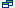Translation(s):

Deutsch: Massen-/Gewichtseinheiten
Français: Unités de masse (poids)
Nederlands: Massa (gewicht) Eenheden

Related publications: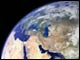Climate Change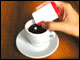AspartameBoron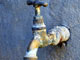Arsenic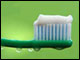Fluoride
• ABC - DEF - GHI - JKL - MNO - PQRS - TUV - WXYZ
•## Get involved!

This summary is free and ad-free, as is all of our content. You can help us remain free and independant as well as to develop new ways to communicate science by becoming a Patron!BECOME A PATRON!

View All